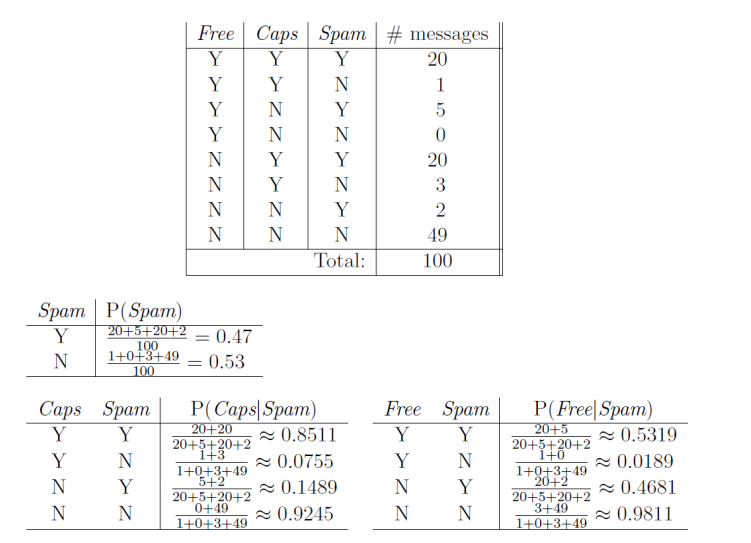# 13 Uncertainty

Outline

A1440显然可以让我按时到达但是我必须在机场过夜……

P(A25能按时到达 | ...) = 0.04；P(A90能按时到达 | ...) = 0.70；P(A120能按时到达 | ... ) = 0.95；P(A1440能按时到达 | ...) = 0.9999

Cavity=false  Toothache=false；Cavity=false  Toothache=true；Cavity=true  Toothache=false；Cavity=true  Toothache=true

0 ≤ P( A ) ≤ 1
P( true ) = 1 and P( false ) = 0
P( A B ) = P( A ) + P( B ) - P( A B )

P(cavity | toothache) = a single number
P(Cavity,Toothache) = 2×2 table summing to 1
P(Cavity | Toothache) = 2-element vector of 2-element vectors

P(a | b) = P(a b) / P(b) if P(b) > 0

0 <= P(a | e) <= 1
P(a1   | e) + P(a2   | e) + ... + P(ak   | e) = 1
P(¬a | e) = 1 - P(a | e)

P (Φ) =∑ω：ω|=Φ   P )
P(toothache) = 0.108+0.012+0.016+0.064 = 0.2
P(cavity ∨ toothache) = 0.108+0.012+0.072+0.008+0.016+0.064=0.28
P(¬ cavity | toothache) = P(¬cavity   toothache) / P(toothache) = (0.016+0.064) / (0.108+0.012+0.016+0.064) = 0.4

P ( Cavity | toothache ) = α P ( Cavity, toothache )
= α [ P ( Cavity, toothache, catch ) + P ( Cavity, toothache , ¬ catch )]
= α [<0.108,0.016> + <0.012,0.064>]
= α <0.12,0.08> = <0.6,0.4>

P ( Y | E = e ) = α P ( Y , E = e ) = α Σ h P ( Y , E = e , H = h )
Y , E , H 构成了域中所有变量的完整集合）

A和B是独立的，假如有
P ( A | B ) = P ( A ) or P ( B | A ) = P ( B ) or P (A, B) = P ( A ) P ( B )
P ( Toothache, Catch, Cavity, Weather ) = P ( Toothache, Catch, Cavity ) P ( Weather )
32 entries reduced to 12; for n independent biased coins, O(2 n ) O(n)

Independence misused

P(A | B, C) = P(A | C)
P(B | A, C) = P(B | C)
P(A, B | C) = P(A | C) P(B | C)

P ( Toothache, Cavity, Catch  有2^3-1=7个独立条目
(1) P ( catch | toothache, cavity ) = P ( catch | cavity )
(2) P ( catch | toothache, ¬ cavity ) = P ( catch | ¬ cavity )

P ( Toothache | Catch, Cavity ) = P ( Toothache | Cavity )
P ( Toothache, Catch | Cavity ) = P ( Toothache | Cavity ) P ( Catch | Cavity )

P ( Toothache, Catch, Cavity )
= P ( Toothache | Catch, Cavity ) P ( Catch, Cavity )
= P ( Toothache | Catch, Cavity ) P ( Catch | Cavity ) P ( Cavity )
= P ( Toothache | Cavity ) P ( Catch | Cavity ) P (Cavity)

=> 贝叶斯法则： P ( a |   b ) = P ( b |   a ) P ( a ) /  P ( b )

P(Y | X) = P(X | Y)P(Y) / P(X) = αP(X | Y)P(Y)

P(Cause | Effect) = P(Effect | Cause)P(Cause) / P(Effect)

P(m | s) = P(s | m)P(m) / P(s) = 0.8*0.0001/0.1 = 0.0008

H = 头疼，F = 患了流感
P(H) = 1/10, P(F) = 1/40, P(H/F) = 1/2

P(F | H) = P(H | F)P(F) / P(H) = 1/8 ≠ P(H | F)

Quiz

P(Cavity | toothache ∧ catch)
= αP(toothache ∧ catch | Cavity) P(Cavity)
= αP(toothache | Cavity) P(catch | Cavity) P(Cavity)

P(Cause, Effect1,..., Effectn) = P(Cause) ∏i P(Effecti | Cause)

P(Toothache, Catch, Cavity)
= P(Toothache | Catch, Cavity) P(Catch, Cavity)
= P(Toothache | Catch, Cavity) P(Catch | Cavity) P(Cavity)
= P(Toothache | Cavity) P(Catch | Cavity) P(Cavity)

1.人类，领域专家
2.更简单的一些概率事实和一些代数，利用链式法则和独立性假设来计算联合分布
3.从数据中学习获得

P(x)=count(x) / total samples

# 14 Bayesian networks

Frequentist vs. Bayesian

“某事发生的概率是0.1”意味着0.1是在无穷多样本的极限条件下能够被观察到的比例

Outline

语法
语义

CS中的概率图模型

混合模型、因子分析、隐马尔科夫模型、卡尔曼滤波器等
系统工程、信息理论、模式识别和统计机制

1.对概率模型框架进行形象化的简单方法
2.模型性质的见解，检查图来保证条件独立性
3.复杂计算，在图像操作中需要推理和学习

For burglary net,1+1+4+2+2=10 numbers (vs. 2^5-1=31)

X: Low pressure  Y:Rain  Z:Traffic
P(x,y,z) = P(x)P(y | x)P(z | y)

P(z | x,y) = P(x,y,z) / P(x,y) = P(x)P(y | x)P(z | y) / P(x)P(y | x) = P(z | y)

Y:Project due  X:Newsgroup busy  Z:Lab full

P(z | x,y) = P(x,y,z) / P(x,y) = P(y)P(x | y)P(z | y) / P(y)P(x | y) = P(z | y)

X:Raining  Z:Ballgame  Y:Traffic
X和Z相互独立么？是的，球赛和下雨导致交通拥堵，这两件事没关系吧

1.Choose an ordering of variables X1,...,Xn
2.For i = 1 to n
select parents from X1,...,Xi-1 such that
P(Xi | Parents(Xi)) = P(Xi | X1,...,Xi-1)

P(X1,...,Xn) = ∏i=1~n P(Xi | X1,...,Xi-1) (链式法则)
= ∏i=1~n P(Xi | Parents(Xi)) (构建)

P(J | M) = P(J)? No
P(A | J,M) = P(A | J)? P(A | J,M) = P(A) ? No
P(B | A,J,M) = P(B | A)? Yes
P(B | A,J,M) = P(B)? No
P(E | B,A,J,M) = P(E | A)? No
P(E | B,A,J,M) = P(E | A,B)? Yes

1+2+4+2+4=13个数字

P(X1,...,Xn) = ∏i=1~n P(Xi | Parents(Xi))

P(B | j,m)公式见课本P437

P(B | j,m)公式见课本P439

P(X1=x1,...,Xn=xn) = P(X1=x1)P(X2=x2 | X1=x1)...P(Xn=xn | X1=x1)
P(Cause,Effect1,...,Effectn) = P(Cause)∏iP(Effecti | Cause)
P(Cause | Effect1,...,Effectn) = P(Effects,Cause) / P(Effects) = αP(Cause,Effects) = αP(Cause)∏iP(Effecti | Cause)

Caps：主题标题是否全部大写
Free：主题标题是否包含词汇"free"，大写或者小写的

Caps = Y ，当且仅当主题标题不含小写字母
Free = Y，当且仅当“”free“出现在主题中（不管大小写）
Spam = Y，当且仅当信息是垃圾邮件
P(Free,Caps,Spam) = P(Spam)P(Caps | Spam)P(Free | Spam)P(Free=Y,Caps=N,Spam=N) = P(Spam=N)P(Caps=N | Spam=N)P(Free=Y | Spam=N) = 0.53*0.9245*0.0189=0.0093

a.准确地解释当给定一组类别已经确定的文档作为“训练数据“时，这样的模型是如何构造的。
b.准确地解释如何对新文档进行分类。
c. 这里独立性假设合理吗？请讨论。

Twennty Newsgroups

– One feature Fij for each grid position <i,j>
– Possible feature values are on / off, based on whether intensity is
more or less than 0.5 in underlying image
– Each input maps to a feature vector
– Here: lots of features, each is binary
Naïve Bayes model:
P(Y | F0,0 ... F15,15) ∝ P(Y) ∏i,j P(Fi,j | Y)

01-07
12-201万+07-10621
12-042752
08-27126
11-103万+
06-125177
01-011693
06-111764
06-112720
04-13276
11-28166
11-28348
01-052万+
12-07293
11-03
07-19
06-13631
12-261957